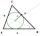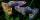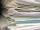# Grade - math word problems

1. Inscribed circleThe circle inscribed in a triangle has a radius 3 cm. Express the area of the triangle using a, b, c.
2. Mother and daughterThe mother is four times older than her daughter. Five years ago, her daughter was seven times younger than her mother. How many years do they have now?
3. RemainderA is an arbitrary integer that gives remainder 1 in the division with 6. B is an arbitrary integer that gives remainder 2 the division by. What makes remainder in division by 3 product of numbers A x B ?
4. Word MATEMATIKAHow many words can be created from the word MATEMATIKA by changing the order of the letters, regardless of whether or not the words are meaningful?
5. DriverI drive at an average speed of 70km/h, I drive 10 thousand km. How long did it take me?
6. GuppiesAudrey has some guppies in a fish tank. The ration of the oranges guppies to silver guppies is 3:5. She has 12y oranges guppies. Write the number of silver guppies she has in terms of y
7. ChordIt is given to a circle k(r=6 cm) and the points A, B such that / AB / = 8 cm lies on k. Calculate the distance of the center of circle S to the midpoint C of the segment AB.
8. Z9–I–4 MO 2017Numbers 1, 2, 3, 4, 5, 6, 7, 8 and 9 were prepared for a train journey with three wagons. They wanted to sit out so that three numbers were seated in each carriage and the largest of each of the three was equal to the sum of the remaining two. The conduct
9. Surface area of the topA cylinder is three times as high as it is wide. The length of the cylinder’s diagonal is 20 cm. Find the surface area of the top of the cylinder.
10. PearsThere were pears in the basket, I took two-fifths of them, and left six in the basket. How many pears did I take?
11. Closest natural numberFind the closest natural number to 4.456 to 44.56 and to 445.6.
12. Iron collectingClass 7A collected 3.2 tonnes of iron more than class 7B. Together they collected 6.4 tonnes of iron to the secondary raw material collection. How much did each class collect?
13. Boys and girlsThere are 28 girls in the hall. 5/7 of all children are boys. How many children and how many boys are there?
14. Functions f,gFind g(1) if g(x) = 3x - x2 Find f(5) if f(x) = x + 1/2Combine like terms 4c+c-7c
16. MO Z9–I–2 - 2017In the VODY trapezoid, VO is a longer base and the diagonal intersection K divides the VD line in a 3:2 ratio. The area of the KOV triangle is 13.5 cm2. Find the area of the entire trapezoid.
17. Rate or interestAt what rate percent will Rs.2000 amount to Rs.2315.25 in 3 years at compound interest?
18. Warmer weatherThis morning it was -6 °C. What temperature did the thermometer show yesterday if it was three times warmer?
19. GroupsIn the 6th class there are 60 girls and 72 boys. We want to divide them into groups so that the number of girls and boys is the same. How many groups can you create? How many girls will be in the group?The ladder is 10 m long The ladder is 8 m high How many meters is the distant heel from the wall?